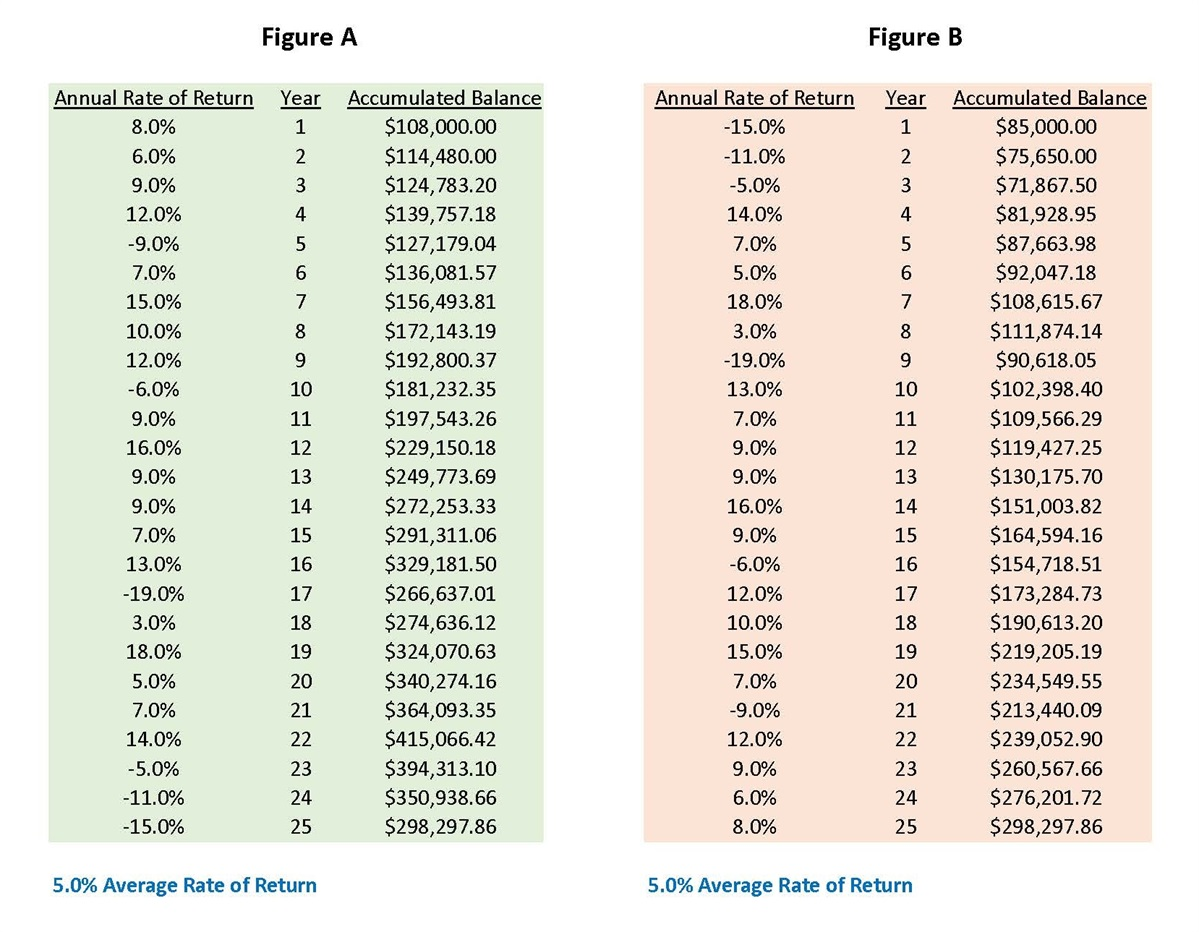## Accumulation Experiences

For Accumulation purposes, let’s assume a lump sum of \$100,000 invested into some sort of asset for 25 years with a hypothetical (Net) 5% Average Rate of Return over that period of time. (Why 5%? - Because in Tim’s experience that has been a predominant Average Rate of Return goal for most folks to shoot for during retirement, and it is easier to use that same return goal to compare the differences between both Accumulation and Distribution experiences for these examples.) However, let’s also inject some realism and assume that the hypothetical Annual Rate of Return will vary from year to year, sometimes negative, but mostly positive to one extent or another. The following is what happens when we use two scenarios with purely hypothetical Annual Rates of Return, but which still equal the same Average Rate of Return of 5%

Figure A - This reflects the results of starting out with a hypothetical positive Annual Rate of Return for the first year and ending with a negative Annual Rate of Return for the last year. However, the Average Rate of Return for the full 25 years was still mathematically 5%. The ending accumulated balance is \$298,297.86.

Figure B - This reflects the results of starting out with a hypothetical negative Annual Rate of Return for the first year and ending with a positive Annual Rate of Return for the last year. However, the Average Rate of Return for the 25 years was still mathematically 5%. The ending accumulated balance is also \$298,297.86.Why? Because the Average Rate of Return for the 25 years is still the same. All we did in this case was reverse the sequence of the Annual Rates of Return (in other words, we flipped it upside down.) Therefore, the ending experience for someone Accumulating this hypothetical asset during this time period using either sequence of returns was exactly the same – though certainly their accumulated balances during the 25 years would have varied widely.

But what happens when you start to Withdrawal funds using the same numbers? Please click on the "Systematic Withdrawals" button below to continue.

This is a hypothetical example and is not representative of any specific investment. Your results may vary